Core Java - Interview Questions and Answers for 'Fibonacci' | Search Java Interview Question - javasearch.buggybread.com# Search Interview QuestionsMore than 3000 questions in repository.There are more than 900 unanswered questions.Have a video suggestion.
Click Correct / Improve and please let us know.
Label / Company      Label / Company / TextSubmit Question

Core Java - Interview Questions and Answers for 'Fibonacci' - 3 question(s) found - Order By Newest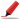Frequently asked in face to face interviews.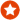Q1. Write a program to print fibonacci series.Core Java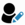Ans. int count = 15;
int[] fibonacci = new int[count];
fibonacci = 0;
fibonacci = 1;
for(int x=2; x < count; x++){
fibonacci[x] = fibonacci[x-1] + fibonacci[x-2];
}

for(int x=0; x< count; x++){
System.out.print(fibonacci[x] + " ");
}Help us improve. Please let us know the company, where you were asked this question :LikeDiscussCorrect / Improveebay   fibonacci series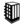Asked in 66 Companies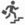basic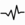frequentRelated Questions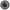What are the steps to be performed while coding Junit with Mocking framework ?What is a Sequence File?What different level of logging you use while coding ?Have you ever encoded the response before sending it back from the service? If Yes , Which encoding was used ?What are the different type of encoding you have used ?How does encoding affect using Reader / writer classes or Stream classes in Java ?What is the difference between html encoding and url encoding ?Does spaces get's encoded in html encoding ?Q2. Write a Program to print fibonacci series using recursion.Core Java2017-08-24 14:41:45Ans. public class FibonacciUsingRecursion {
public static void main(String[] args) throws IOException{
getNextFibanocci(1,1);
}

static int getNextFibanocci(int a,int b){
if(a+b >= 100){
return 0;
} else {
System.out.println(a+b);
getNextFibanocci(b,a+b);
}

return 0;
}
}Help us improve. Please let us know the company, where you were asked this question :LikeDiscussCorrect / Improvefibonacci  recursionRelated QuestionsWhat will happen if we don't have termination statement in recursion ?Find Fibonacci first n numbers using recursion ?Write an Algorithm for Graph Traversal ? The Graph has a loop.Write a Program to print factorial of a number using recursionWrite a program to print sum of numbers between the start and end number

For example - Passing start number as 2 and end number as 10, it should print 2+3+4+5+6+7+8+9+10 = 54Write a program using Recursion to print multiplication of numbers between the start and end number

For example - Passing start number as 2 and end number as 10, it should print 2*3*4*5*6*7*8*9*10 = 3628800What is recursion in Java ?What will happen if we don't have termination statement in recursion ?Which of the two - iteration or recursion - is slower ?Very Frequently asked.Q3. Write a program to print fibonacci series.Ans. int count = 15;
int[] fibonacci = new int[count];
fibonacci = 0;
fibonacci = 1;
for(int x=2; x < count; x++){
fibonacci[x] = fibonacci[x-1] + fibonacci[x-2];
}

for(int x=0; x< count; x++){
System.out.print(fibonacci[x] + " ");
}Help us improve. Please let us know the company, where you were asked this question :LikeDiscussCorrect / ImprovefibonacciAsked in 4 CompaniesbasicfrequentRelated QuestionsDifference between == and .equals() ?Why is String immutable in Java ?Explain the scenerios to choose between String , StringBuilder and StringBuffer ?

or

What is the difference between String , StringBuilder and StringBuffer ?What are the difference between composition and inheritance in Java?Does garbage collection guarantee that a program will not run out of memory?What are different ways to create String Object? Explain.Why Char array is preferred over String for storing password?Why do we need Inner classes ? Cant we just work with outer classes wherever we implement Inner classes ?What is the difference between declaration, instantiation and initialization ?Explain OOPs

or

Explain OOPs Principles

or

Explain OOPs Concepts

or

Explain OOPs features

or